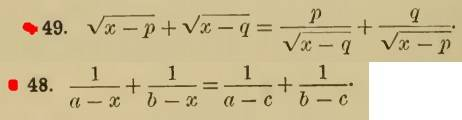1. Homework Statement

## The Attempt at a Solution

I just want some clarification about the rules on solving quadratic equation. My question is coming from my solution for problem 49.

Solving problem 49 [/B]
Factor: (x−p−q)(√(x−q)+√(x−p))=0
Therefore: x−p−q=0⇒x=p+q this agrees with the answer in my book

What Im confused about is the part where I divided out the lhs by (√(x−q)+√(x−p)). This factor contains x in it and I'm worried that I may have discarded the other root for the equation. And since it is a quadratic equation I am expecting to get two roots for the equation. Can you enlighten me about this matter? Thanks.

ehild
Homework Helper1. Homework Statement

## The Attempt at a Solution

I just want some clarification about the rules on solving quadratic equation. My question is coming from my solution for problem 49.

Solving problem 49 [/B]
Factor: (x−p−q)(√(x−q)+√(x−p))=0
Therefore: x−p−q=0⇒x=p+q this agrees with the answer in my book

What Im confused about is the part where I divided out the lhs by (√(x−q)+√(x−p)). This factor contains x in it and I'm worried that I may have discarded the other root for the equation. And since it is a quadratic equation I am expecting to get two roots for the equation. Can you enlighten me about this matter? Thanks.
When you solve an equation of form f(x) g(x) =0, you use the fact that the product is zero if either f(x)=0 or g(x)=0. In principle, it is possible that √(x−q)+√(x−p) = 0. What does it mean for √(x−q) and √(x−p)?
You started the solution by multiplying the whole equation with √(x−q)√(x−p). What is the condition that you may do it?

The one and only posibility that √(x−q)+√(x−p) =0 is if x=p=q. Therefore x=q=p is also a solution? Im still confused. Please bear with me.

Ray Vickson
Homework Helper
Dearly Missed
The one and only posibility that √(x−q)+√(x−p) =0 is if x=p=q. Therefore x=q=p is also a solution? Im still confused. Please bear with me.

You are not allowed to have either ##x = p## or ##x = q##, because the right-hand-side of your original equation would then contain division by zero, which is absolutely forbidden.

You are not allowed to have either ##x = p## or ##x = q##, because the right-hand-side of your original equation would then contain division by zero, which is absolutely forbidden.

What? When x = x+p we will also have division by zero right? Why is it that √(x-q)+√(x-p) is not allowed to be equal zero but x-p-q can be equal zero?

SammyS
Staff Emeritus
Homework Helper
Gold Member
What? When x = x+p we will also have division by zero right? Why is it that √(x-q)+√(x-p) is not allowed to be equal zero but x-p-q can be equal zero?
The following doesn't make sense unless p = 0 .
When x = x+p

Homework Helper
Gold Member
Suggestion: Group the sqrt (x-p ) terms on one side of the equation (one term will be in the denominator), and the sqrt(x-q) terms on the other side of the equation and square both sides of the equation. You can then do some additional algebra. It's then a couple more steps, but you do get a quadratic expression for x in terms of p and q. The quadratic expression does factor and simplify and it does have a simple solution.

Last edited:
ehild
Homework Helper
The one and only posibility that √(x−q)+√(x−p) =0 is if x=p=q. Therefore x=q=p is also a solution? Im still confused. Please bear with me.
You started the solution with multiplying the whole equation by √(x−q)*√(x−p). Before multiplying an equation with an expression you have to exclude that it is zero.

Ray Vickson
Homework Helper
Dearly Missed
What? When x = x+p we will also have division by zero right? Why is it that √(x-q)+√(x-p) is not allowed to be equal zero but x-p-q can be equal zero?

$$\text{original right-hand-side} = \frac{q}{\sqrt{x-p}} + \frac{p}{\sqrt{x-q}}$$
If you take ##x = p## or ##x = q## you will have ##1/\sqrt{0}## on the right, and that is forbidden.

Of course, after you multiply your equation by ##\sqrt{x-p} \sqrt{x-q}## you would have
$$\text{new right-hand-side} =q \sqrt{x-q} + p \sqrt{x-p}$$
and in that new rhs you are certainly allowed to have ##x = p## or ##x = q##. However, that would not be giving you a solution to the original equation.

Last edited:
$$\text{original right-hand-side} = \frac{1}{\sqrt{x-p}} + \frac{1}{\sqrt{x-q}}$$
$$\text{new right-hand-side} = \sqrt{x-q} + \sqrt{x-p}$$